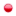###Author Topic: RAND function in Formula node  (Read 341 times)

#### Flint Stryker##### RAND function in Formula node
The limitation of the Parameter node configured as a RNG is that I can't get multiple random numbers from it. I need to be able to get several random numbers from a single seed. Even better would be a Random calculation node that can give a random number between two values, but I would be happy with a simple RAND function in the Formula node. A RAND Formula nodes would give me all the random values I need.
« Last Edit: December 28, 2020, 08:55:08 PM by seversky »

#### Alexis Vaisse

••• Posts: 187##### Re: RAND function in Formula node
Hi,

Thanks a lot for the suggestion.
We'll add a RAND function for the Formula node in the next release of Instant Terra.

Also note that's it's possible to generate random numbers by using a Python node: create a new custom node of type 'Python', edit the graph of the node, set one value output node named Output and use a script such as:

from random import random
Output=random()